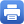Columbia Home
Lie Groups and Representations

This course is taken in sequence, part 1 in the fall, and part 2 in the spring.

### Lie Groups and Representations I

I Basic Notions

• Abstract groups, algebraic groups over a field, topological groups, Lie groups
• Subgroups, normal subgroups, quotient groups
• Homomorphisms of groups – image, kernel, exact sequences
• Cyclic groups, abelian groups, nilpotent groups
• Conjugacy classes, left and right cosets of a subgroup

II Algebraic Examples

• Units of a ring, k* for k a field, roots of unity in a commutative ring, R*, S1 in C*
• GL(n, R) as the group of units of n x n-matrices over a commutative ring
• The determinant and SL(n, R), O(n, R), Sympl(2n, R) when there is (-1) in R
• Algebraic groups of the above types over a field, definition of linear algebraic groups
• Group structure on an elliptic curve
• Group of p-adic integers, and its multiplicative group of units

III Geometric Examples and Symmetry

• Permutation groups
• Symmetries of regular plane figures and of Platonic solids
• The Lie groups SL(n, R), SO(n, R), SO(p, q), Sympl(2n, R)
• Isometries of the line, the plane, and higher dimensional Euclidean spaces
• Isometries of spheres and of Minkowski space. The Poincaré group
• Isometries of the hyperbolic plane, conformal isomorphisms of S2, relation with SL(2, R) and SL(2, C)
• Clifford algebras and the spin groups
• The Heisenberg group

IV Lie Algebras

• Definition, examples of the Lie algebra of an associative algebra
• The Lie algebra of a Lie group. The universal enveloping algebra and the Poincaré-Birkhoff-Witt theorem

V Representations

• Definition in the various categories of groups, representations of a Lie algebra
• Infinitesimal generators for the action of a Lie group
• The infinitesimal representation associated to a linear representation of a Lie group
• Turning actions into linear representations on the functions
• Classification of the (finite dimensional) representations of sl(2, C), SU(2), and SO(3)
• Representations of the Heisenberg algebra

VI Representations of Finite and Compact Lie Groups

• Complete reducibility, Schur’s lemma, characters, orthogonality relations for characters of a finite group
• Dimension of the space of characters of a finite group
• The decomposition of the regular representation of a finite group
• Characters of a compact group – complete reducibility, Schur’s lemma, orthogonality of characters
• Peter-Weyl theorem (except the proof of the decomposibility of a Hilbert space representation into finite dimensional sub representations)
• Example of L2(S1) and Fourier analysis
• Example of L2(S2) as a module over SO(3) and spherical harmonics

VII Finite Groups and Counting Principles

• Orders of elements and subgroups
• Groups of order pn are nilpotent
• Subgroups of index 2 are normal
• The Sylow theorems
• Classification of groups of order pq for p, q distinct primes. Groups of order 12

### Lie Groups and Representations II

I Lie Groups and Lie Algebras: the Exponential Mapping

• Baker-Campbell-Hausdorff formula
• A Lie group is determined by its Lie algebra up to covering
• Action of a Lie group is determined by its infinitesimal action

II Maximal Tori of a Compact Lie Group

• Existence and uniqueness up to conjugation
• Every element is contained in a maximal torus
• Regular elements
• The Weyl group
• Weyl group action on the maximal torus and on corresponding abelian Lie algebra
• Decomposition of the adjoint representation root spaces. Weyl chambers
• Groups generated by reflection
• Positive roots, dominant root and alcove
• Dynkin diagrams
• The classical examples SU(n), SO(n), Sympl(2n)

III Complex Semi-Simple Lie Groups and Lie Algebras
IV Irreducible Representations of Compact Groups

• Weight spaces, dominant weights
• Examples for SU(n), Sympl(2n) and SO(n)

V Selected Topics, chosen from

• Borel-Weil-Bott theory
• Infinite-dimensional representations of SL(2, R)
• Kac-Moody algebras
• The Virasoro algebra
• SupersymmetryPrint this page